# 12 Results

View
Selected filters:
• Rectangle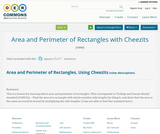Unrestricted Use
CC BY
Rating

This is a lesson for learning about area and perimeter of rectangles.

This corresponds to "College and Career Ready" standard (3.MD.7a) -- Find the area of a rectangle with whole-number side lengths by tiling it, and show that the area is the same as would be found by multiplying the side lengths.
(I was not able to find that standard here.)

Subject:
Mathematics
Material Type:
Lesson Plan
Author:
Susan Jones
01/28/2017
Remix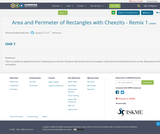Unrestricted Use
CC BY
Rating

This is a hands-on guided lesson for relating area to factors. Students will model area of rectangles using Cheez-Its and define factors as the dimensions of the rectangles.

Subject:
Mathematics
Material Type:
Lesson Plan
Author:
Donna Parrish
08/08/2019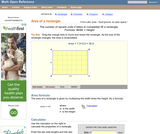Rating

A web page and interactive applet showing the ways to calculate the area of a rectangle. The user can drag the vertices of the rectangle and the other points change automatically to ensure it remains a rectangle. A grid inside the shape allows students to estimate the area visually, then check against the actual computed area. The text on the page gives three different ways to calculate the area with a formula for each. The applet uses one of the methods to compute the area in real time, so it changes as the rectangle is reshaped with the mouse. A companion page is http://www.mathopenref.com/rectangle.html showing the definition and properties of a rectangle Applet can be enlarged to full screen size for use with a classroom projector. This resource is a component of the Math Open Reference Interactive Geometry textbook project at http://www.mathopenref.com.

Subject:
Geometry
Material Type:
Simulation
Provider:
Math Open Reference
Author:
John Page
02/16/2011Only Sharing Permitted
CC BY-NC-ND
Rating

Subject:
Geometry
Material Type:
Lesson Plan
Author:
summer dickerhoof
12/07/2017
Remix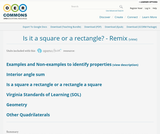Conditional Remix & Share Permitted
CC BY-NC
Rating

Students will look at examples and non-examples of squares and rectangles to determine properties of the two shapes. Students practice drawing the shapes with specific dimensions and building up to is a square also a rectangle and is a rectangle also a square?

Subject:
Mathematics
Material Type:
Activity/Lab
Lesson Plan
Author:
Tres Wells
08/08/2019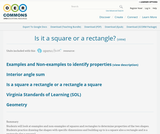Conditional Remix & Share Permitted
CC BY-NC
Rating

Students will look at examples and non-examples of squares and rectangles to determine properties of the two shapes. Students practice drawing the shapes with specific dimensions and building up to is a square also a rectangle and is a rectangle also a square?

Subject:
Mathematics
Material Type:
Activity/Lab
Lesson Plan
Author:
Peter Fiddner
02/13/2016Conditional Remix & Share Permitted
CC BY-NC-SA
Rating

In this activity, learners slide shapes to create unusual tiled patterns. Learners transform a rectangle into a more interesting shape and then make a tessellation by repeating that shape over and over again. Learners will also calculate the area of a rectangle. This activity works best as a "centers" activity.

Subject:
Geometry
Material Type:
Activity/Lab
Provider:
Exploratorium
Author:
Exploratorium
Gordon and Betty Moore Foundation
National Science Foundation
The Exploratorium
12/07/2010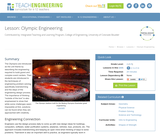Educational Use
Rating

The lesson begins by introducing Olympics as the unit theme. The purpose of this lesson is to introduce students to the techniques of engineering problem solving. Specific techniques covered in the lesson include brainstorming and the engineering design process. The importance of thinking out of the box is also stressed to show that while some tasks seem impossible, they can be done. This introduction includes a discussion of the engineering required to build grand, often complex, Olympic event centers.

Subject:
Architecture and Design
Engineering
Education
Mathematics
Geometry
Material Type:
Activity/Lab
Lesson Plan
Provider:
TeachEngineering
Provider Set:
TeachEngineering
Author:
Abigail Watrous
Denali Lander
Janet Yowell
Katherine Beggs
Melissa Straten
Tod Sullivan
09/18/2014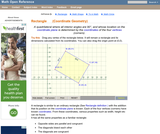Rating

An interactive applet and associated web page that show the definition and properties of a rectangle in coordinate geometry. The applet has a rectangle with draggable vertices. As the user re-sizes the rectangle the applet continuously recalculates its width, height and diagonals from the vertex coordinates. Rectangle can be rotated on the plane to show the more complex cases. The grid, coordinates and calculations can be turned on and off for class problem solving. The applet can be printed in the state it appears on the screen to make handouts. The web page has a full definition of a rectangle when the coordinates of the points defining it are known, and has links to other pages relating to coordinate geometry. Applet can be enlarged to full screen size for use with a classroom projector. This resource is a component of the Math Open Reference Interactive Geometry textbook project at http://www.mathopenref.com.

Subject:
Geometry
Material Type:
Simulation
Provider:
Math Open Reference
Author:
John Page
02/16/2011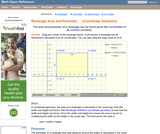Rating

An interactive applet and associated web page showing how to find the area and perimeter of a rectangle from the coordinates of its vertices. The rectangle can be either parallel to the axes or rotated. The grid and coordinates can be turned on and off. The area and perimeter calculation can be turned off to permit class exercises and then turned back on the verify the answers. The applet can be printed as it appears on the screen to make handouts. The web page has a full description of the method for determining area and perimeter, a worked example and has links to other pages relating to coordinate geometry. Applet can be enlarged to full screen size for use with a classroom projector. This resource is a component of the Math Open Reference Interactive Geometry textbook project at http://www.mathopenref.com.

Subject:
Geometry
Material Type:
Simulation
Provider:
Math Open Reference
Author:
John Page
02/16/2011Educational Use
Rating

In this Cyberchase video segment, the CyberSquad learns what happens to the area of a rectangle when the shape enclosed by the perimeter changes.

Subject:
Mathematics
Geometry
Material Type:
Lecture
Provider:
PBS LearningMedia
Provider Set:
PBS Learning Media: Multimedia Resources for the Classroom and Professional Development
Teachers' Domain
Author:
U.S. Department of Education
WNET
07/08/2008
Remix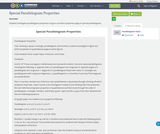Unrestricted Use
CC BY
Rating

Students investigate parallelogram properties to figure out which properties apply to special parallelograms.

Subject:
Mathematics
Material Type:
Activity/Lab
Author:
Becky Lancaster